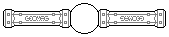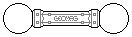Web textodigital.com
Pseudogeodesic sphere to scale 1
If we want to stabilize a regular dodecahedron without using panels, the obvious solution is building pentagonal pyramids on each face. However, if we build them pointing towards the center of the object (left-hand picture), the rods make angles which are too acute at the vertices, and so they cannot make appropriate contact (right-hand picture).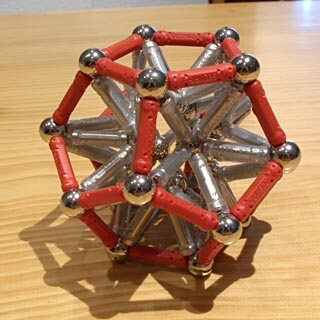Regular dodecahedron with internal pentagonal pyramids 122 pieces: 32 balls, 90 rods (698.20 g)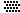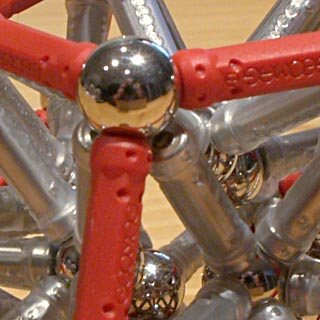Detail: vertex of the regular dodecahedron with internal pentagonal pyramids
The problem disappears if we build the pyramids pointing outwards. But, in that case, the edges of the polyhedron are nearly hidden, and we only recognize the object because of the rod colors. It cannot even rest over any of its faces: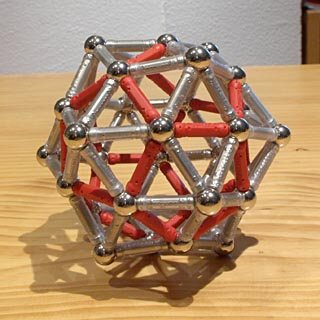Regular dodecahedron with external pentagonal pyramids 122 pieces: 32 balls, 90 rods (698.20 g)If we build the regular dodecahedron to double scale, the vertex angles remain the same, which requires beginning to build the pyramids outwards. However, the pyramids have two levels now, and the second one can be turned inwards. So, the original object turns out to be less hidden, and we get single-scale pentagons which are parallel to the faces: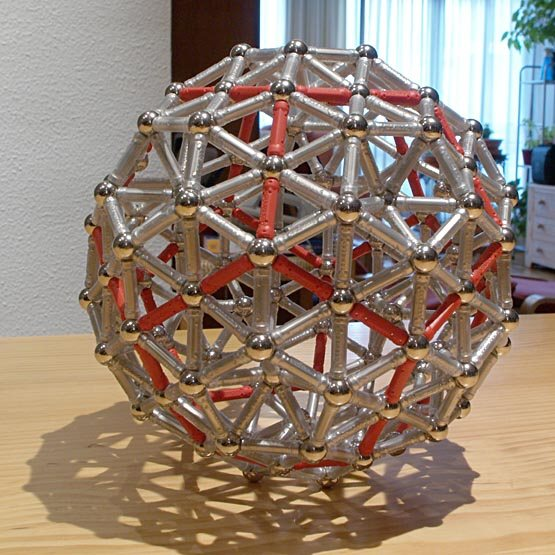Double-scale regular dodecahedron 482 pieces: 122 balls, 360 rods (2.74 kg)By just changing the rod colors, the double-scale regular dodecahedron seems a different object:Pseudogeodesic sphere 482 pieces: 122 balls, 360 rods (2.74 kg)The «new» object is made of 12 pentagons and 30 hexagons, and it must not be mistaken with the truncated regular icosahedron, which is made of 12 pentagons and 20 hexagons. It is not an Archimedean solid, as it has two different types of vertices: 60 are made of one pentagon and two hexagons, and 20 are made of three hexagons. Archimedean solids have all their vertices equal, among other properties.
But we have an additional «little» problem in the previous paragraph. The vertex angle of a regular hexagon is 120 degrees, which means that, when three regular hexagons join in a vertex, their angles add up to 360 degrees, a full circle, and that vertex will be planar, that is, it will not be a vertex at all.
By considering the object as made of 30 hexagons and 12 pentagons, however, it is very near to what is called a two-frequency dodecahedral geodesic sphere. Indeed, the triangle structures are the same in both objects, just all the vertices of the true geodesic sphere are moved to lay on the surface of a sphere, which requires slightly modifying the length of every edge. Of course, we cannot do that to Geomag rods, which is why we have to call this object a pseudogeodesic sphere. For us, this will be the pseudogeodesic sphere to scale 1.
In case you were wondering: a one-frequency dodecahedral geodesic sphere is not more than a regular dodecahedron with external pyramids on its faces, as we built above, but with the apices of the pyramids moved to the same sphere on which the dodecahedron vertices lay. Again, that kind of transformations are not for Geomag, and the untransformed object is not round enough to be called a sphere at all.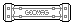Perhaps you noticed the green rods in the previous picture. Those two rods in each pyramid are perfectly horizontal, and another five upside-down pyramids could be built with them as part of their bases, all of which lay on the same horizontal plane: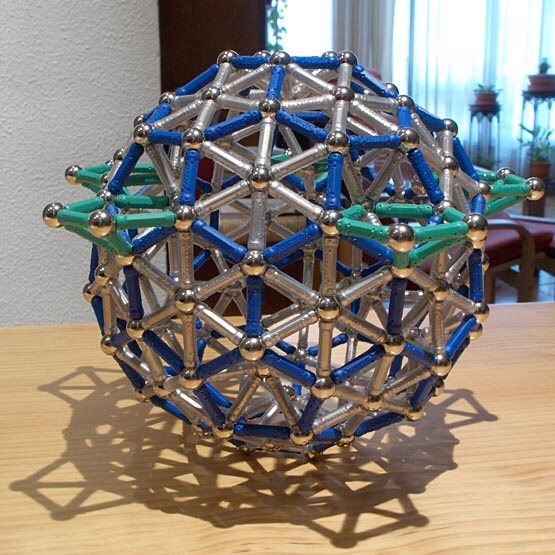Pseudogeodesic sphere: common plane of the pyramids
We could build a regular icosahedron over each of these pyramids: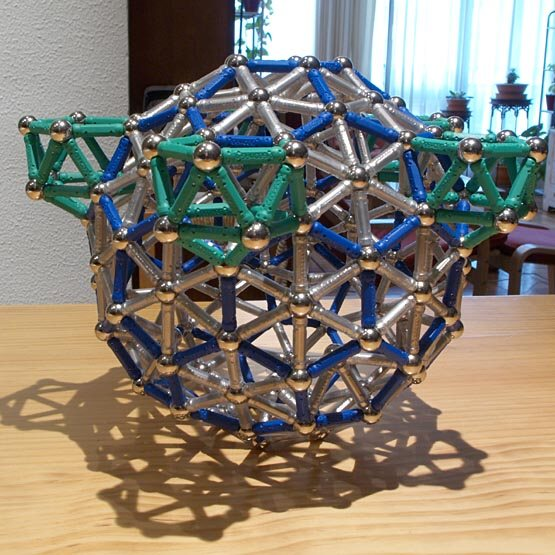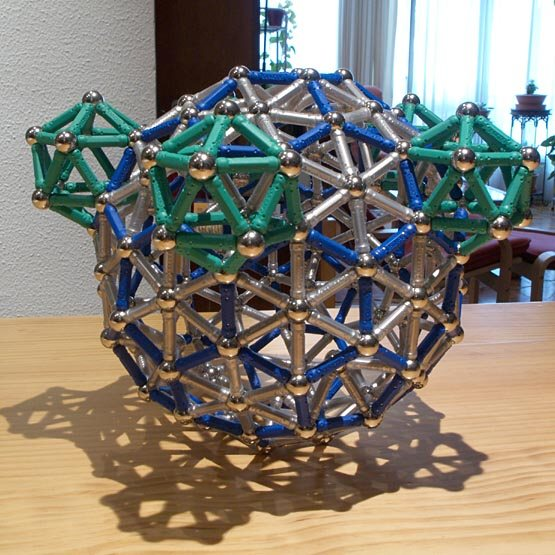Pseudogeodesic sphere with five icosahedra
We could also add several pentagonal prisms to each icosahedron (here we added two of them), so that the five structures they form could be supports or legs for the sphere (of course, after we turn it upside down):Pseudogeodesic sphere over five pentagonal supports 797 pieces: 197 balls, 535 rods, 15 pentagons, 50 squares (4.34 kg)